## Bartlett Function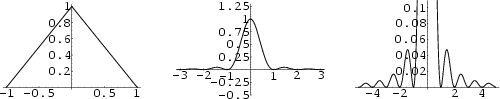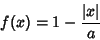(1)

which is a generalization of the one-argument Triangle Function. Its Full Width at Half Maximum is. It has Instrument Function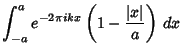(2)

Letting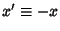in the first part therefore gives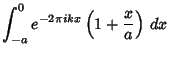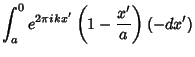(3)

Rewriting (2) using (3) gives(4)

Integrating the first part and using the integral(5)

for the second part gives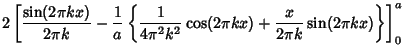(6)

whereis the Sinc Function. The peak (in units of) is 1. The function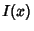is always positive, so there are no Negative sidelobes. The extrema are given by lettingand solving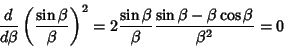(7)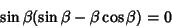(8)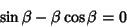(9)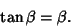(10)

Solving this numerically givesfor the first maximum, and the peak Positive sidelobe is 0.047190. The full width at half maximum is given by settingand solving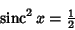(11)

for, yielding(12)

Therefore, with,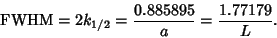(13)

Bartlett, M. S. Periodogram Analysis and Continuous Spectra.'' Biometrika 37, 1-16, 1950.